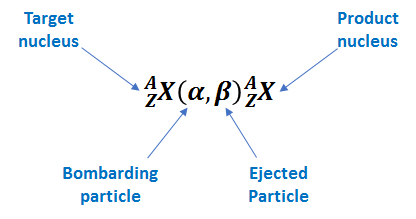# Problem: Determine the missing species in these transmutations, and write a full nuclear equation from the shorthand notation:  (c) __ (α,2n) 244Cf

###### FREE Expert Solution

We’re being asked to determine the missing species in the given nuclear transmutation and write the full nuclear equation from the shorthand notation.

Nuclear transmutation is changing one nuclide (chemical element) or an isotope into another chemical element.

A shorthand notation is used to represent nuclear transmutations lists the target nucleus, the bombarding particle and the ejected particle in parentheses, then the product nucleus.Given: __ (α,2n) 244Cf

*let’s use X to represent the missing species

89% (280 ratings)###### Problem Details

Determine the missing species in these transmutations, and write a full nuclear equation from the shorthand notation:

(c) __ (α,2n) 244Cf